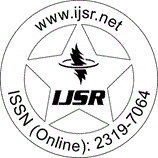International Journal of Science and Research (IJSR)
Call for Papers | Fully Refereed | Open Access | Double Blind Peer Reviewed

Research Paper | Mathematics | India | Volume 3 Issue 5, May 2014

# Shape Control of the Rational Quadratic Trigonometric B?zier Curve with two Shape Parameters

Mridula Dube  | Bharti Yadav

Abstract: A rational quadratic trigonometric Bzier curve with two shape parameters; which is analogous to cubic Bzier curve; is presented in this paper. The shape of the curve can be adjusted as desired; by simply altering the value of shape parameters; without changing the control polygon. The rational quadratic trigonometric Bzier curve can be made close to the rational cubic Bzier curve or closer to the given control polygon than the rational cubic Bzier curve. The ellipses and circles can be representation exactly by using rational quadratic trigonometric Bzier curve.

Keywords: Rational Trigonometric Bzier Basis Function, Rational Trigonometric Bzier Curve, Shape Parameter, Open curves, Close curves

Edition: Volume 3 Issue 5, May 2014,

Pages: 1019 - 1023